SSC BOARD PAPERS IMPORTANT TOPICS COVERED FOR BOARD EXAM 2024

### Practice Set 5.2, Chapter 5 - Quadrilaterals Mathematics Part II Solutions for Class 9 Math

Practice Set 5.2
###### Practice Set 5.2
Question 1.

In figure 5.22, 􀀍ABCD is a parallelogram, P and Q are midpoints of side AB and DC respectively, then prove 􀀍APCQ is a parallelogram.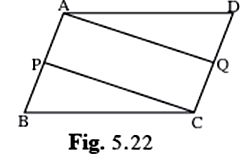Given AB ∥ to DC and AB = DC as ABCD is ∥gram.

⇒ AP ∥CQ (parts of ∥ sides are ∥) & 1/2 AB = 1/2 DC

⇒ AP = QC (P and Q are midpoint of AB and DC respectively)

⇒ AP = PB and DQ = QC

Hence APCQ is a parallelogram as the pair of opposite sides is = and ∥.

Question 2.

Using opposite angles test for parallelogram, prove that every rectangle is a parallelogram.

Opposite angle property of parallelogram says that the opposite angles of a parallelogram are congruent.

Given a rectangle which had at least one angle as 90°.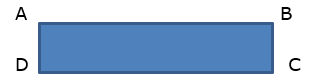If ∠ A is 90° and AD = BC (opposite sides of rectangle are ∥ and =)

AB is transversal

⇒ ∠ A + ∠B = 180 (angles on the same side of transversal is 180°)

But ∠B + ∠C is 180 (AD ∥ BC, opposite sides of rectangle)

⇒ ∠ A = ∠C = 90°

Since opposite ∠s are equal this rectangle is a parallelogram too.

Question 3.

In figure 5.23, G is the point of concurrence of medians of ΔDEF. Take point H on ray DG such that D-G-H and DG = GH, then prove that 􀀍GEHF is a parallelogram.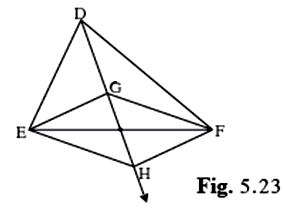Given G is the point of concurrence of medians of Δ DEF so the medians are divided in the ratio of 2:1 at the point of concurrence. Let O be the point of intersection of GH AND EF.

The figure is shown below: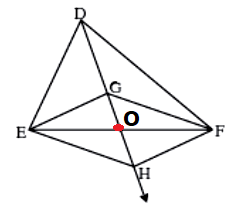⇒ DG = 2 GO

But DG = GH

⇒ 2 GO = GH

Also DO is the median for side EF.

⇒ EO = OF

Since the two diagonals bisects each other

⇒ GEHF is a ∥gram.

Question 4.

Prove that quadrilateral formed by the intersection of angle bisectors of all angles of a parallelogram is a rectangle. (Figure 5.24)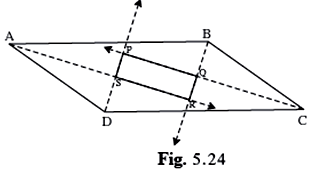Given ABCD is a parallelogram

AR bisects ∠BAD, DP bisects ∠ADC , CP bisects ∠BCD and BR bisects ∠CBA

1/2 ∠ABC = ∠RBA

∠BAR + ∠RBA = 1/2 × 180° = 90°

⇒ Δ ARB is right angled at ∠ R since its acute interior angles are complementary.

Similarly Δ DPC is right angled at ∠ P and

Also in Δ COB , ∠BOC = 90° ⇒ ∠POR = 90° (vertically opposite angles)

Similarly in ΔADS , ∠ASD = 90° = ∠PSR (vertically opposite angles)

Since vertically opposite angles are equal and measures 90° the quadrilateral is a rectangle.

Question 5.

In figure 5.25, if points P, Q, R, S are on the sides of parallelogram such that AP = BQ = CR = DS then prove that 􀀍PQRS is a parallelogram.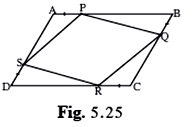Given ABCD is a parallelogram so

and DC = AB and DC ∥ AB

also AP = BQ = CR = DS

⇒ AS = CQ and PB = DR

in ΔAPS and Δ CRQ

∠ A = ∠C (opposite ∠s of a parallelogram are congruent)

AS = CQ

AP = CR

ΔAPS ≅ Δ CRQ( SAS congruence rule)

⇒ PS = RQ (c.p.c.t.)

Similarly PQ= SR

Since both the pair of opposite sides are equal

PQRS is ∥gram.